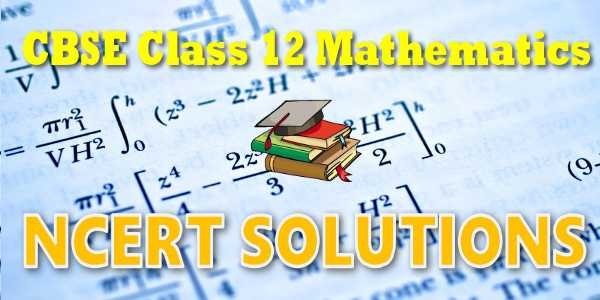No products in the cart.

# CBSE - Class 12 - Mathematics - Differential Equations - NCERT Solutions## CBSE, JEE, NEET, NDA

Question Bank, Mock Tests, Exam Papers

NCERT Solutions, Sample Papers, Notes, Videos

## NCERT Solutions for Mathematics Differential Equations## myCBSEguide App

Complete Guide for CBSE Students

NCERT Solutions, NCERT Exemplars, Revison Notes, Free Videos, CBSE Papers, MCQ Tests & more.

# NCERT solutions for class 12 Mathematics## NCERT Class 12 Mathematics Chapter-wise Solutions

### Maths Part I

1. Chapter 1 – Relations and Functions
2. Chapter 2 – Inverse Trigonometric Functions
3. Chapter 3 – Matrices
4. Chapter 4 – Determinants
5. Chapter 5 – Continuity and Differentiability
6. Chapter 6 – Application of Derivatives

### Maths Part II

1. Chapter 7 – Integrals
2. Chapter 8 – Application of Integrals
3. Chapter 9 – Differential Equations
4. Chapter 10 – Vector Algebra
5. Chapter 11 – Three Dimensional Geometry
6. Chapter 12 – Linear Programming
7. Chapter 13 – Probability

## CBSE class 12th Mathematics have two books. Each book has chapters and topics.

1. NCERT Mathematics Book Class 12 Part-1
2. NCERT Mathematics Book Class 12 Part-2

Here is the list of topics covered under each chapter of class 12 Mathematics NCERT text book.

### Relations and Functions

• 1.1 Introduction
• 1.2Types of Relations
• 1.3Types of Functions
• 1.4Composition of Functions and Invertible Function
• 1.4Binary Operations

### 2. Inverse Trigonometric Functions

• 2.1 Introduction
• 2.2 Basic Concepts
• 2.3 Properties of Inverse Trigonometric Functions

### 3. Matrices

• 3.1 Introduction
• 3.2 Matrix
• 3.3 Types of Matrices
• 3.4 Operations on Matrices
• 3.5 Transpose of a Matrix
• 3.6 Symmetric and Skew Symmetric Matrices
• 3.7 Elementary Operation (Transformation) of a Matrix
• 8 Invertible Matrices

### 4. Determinants

• 4.1 Introduction
• 4.2 Determinant
• 4.3 Properties of Determinants
• 4.4 Area of a Triangle
• 4.5 Minors and Cofactors
• 4.6 Adjoint and Inverse of a Matrix
• 4.7 Applications of Determinants and Matrices

### 5. Continuity and Differentiability

• 5.1 Introduction
• 5.2 Continuity
• 5.3 Differentiability
• 5.4 Exponential and Logarithmic Functions
• 5.5 Logarithmic Differentiation
• 5.6 Derivatives of Functions in Parametric Forms
• 5.7 Second Order Derivative
• 5.8 Mean Value Theorem

### 6. Application of Derivatives

• 6.1 Introduction
• 6.2 Rate of Change of Quantities
• 6.3 Increasing and Decreasing Functions
• 6.4 Tangents and Normals
• 6.5 Approximations
• 6.6 Maxima and Minima

### 7.Integrals

• 7.1 Introduction
• 7.2 Integration as an Inverse Process of Differentiation
• 7.3 Methods of Integration
• 7.4 Integrals of some Particular Functions
• 7.5 Integration by Partial Fractions
• 7.6 Integration by Parts
• 7.7 Definite Integral
• 7.8 Fundamental Theorem of Calculus
• 7.9 Evaluation of Definite Integrals by Substitution
• 7.10 Some Properties of Definite Integrals

### 8. Application of Integrals

• 8.1 Introduction
• 8.2 Area under Simple Curves

### 9.Differential Equations

• 9.1 Introduction
• 9.2 Basic Concepts

### 10  Vector Algebra

• 10.1 Introduction
• 10.2 Some Basic Concepts
• 10.3 Types of Vectors
• 10.5 Multiplication of a Vector by a Scalar
• 10.6 Product of Two Vectors

### 12.Linear Programming

• 12.1 Introduction
• 12.2 Linear Programming Problem and its Mathematical Formula
• 12.3 Different Types of Linear Programming Problems

### 13. Probability

• 13.1 Introduction
• 13.2 Conditional Probability
• 13.3 Multiplication Theorem on Probability
• 13.4 Independent Events
• 13.5 Bayes' Theorem
• 13.6 Random Variables and its Probability Distributions
• 13.7 Bernoulli Trials and Binomial Distribution

## NCERT Solutions Chapter 9 Differential Equations

In Class XI and in Chapter 5 of the present book, we discussed how to differentiate a given function f with respect to an independent variable, i.e., how to find f ′(x) for a given function f at each x in its domain of definition. Further, in the chapter on Integral Calculus, we discussed how to find a function f whose derivative is the function g, which may also be formulated as follows: For a given function g, find a function f such that) An equation of the form (1) is known as a differential equation. A formal definition will be given later. These equations arise in a variety of applications, may it be in Physics, Chemistry, Biology, Anthropology, Geology, Economics etc. Hence, an indepth study of differential equations has assumed prime importance in all modern scientific investigations. In this chapter, we will study some basic concepts related to differential equation, general and particular solutions of a differential equation, formation of differential equations, some methods to solve a first order - first degree differential equation and some applications of differential equations in different areas.

## NCERT Solutions for Class 12th Mathematics

NCERT Solutions Class 12 Mathematics PDF (Download) Free from myCBSEguide app and myCBSEguide website. Ncert solution class 12 Mathematics includes text book solutions from both part 1 and part 2. NCERT Solutions for CBSE Class 12 Mathematics have total 13 chapters. Class 12 Mathematics ncert Solutions in pdf for free Download are given in this website. Ncert Mathematics class 12 solutions PDF and Mathematics ncert class 12 PDF solutions with latest modifications and as per the latest CBSE syllabus are only available in myCBSEguide.

## NCERT Solutions for Class 3## myCBSEguide

Trusted by 1 Crore+ Students

#### Question Paper Creator

• Create papers in minutes
• Print with your name & Logo